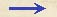Discorsi Propositions 3/10-th-07 Discorsi Proposition3/10-th-07THEOREMA, PROPOSITIO X. THEOREM. PROPOSITION XImpetus seu momentum cuiuslibet semiparabolae aequatur momento naturaliter cadentis in perpendiculari ad horizontem, quae tanta sit quanta est composita ex sublimitate cum altitudine semiparabolae. The momentum [impetus seu momentum] acquired by a particle at the terminal point of any semi-parabola is equal to that which it would acquire in falling through a vertical distance equal to the sum of the sublimity and the altitude of the semi-parabola. Sit semiparabola ab, cuius sublimitas da, altitudo vero ac, ex quibus {10} componitur perpendicularis dc: dico, impetum semiparabolae in b esse aequalem momento naturaliter descendentis ex d in c. Ponatur ipsamet dc mensura esse temporis et impetus, et accipiatur media proportionales inter cd, da, cui aequales ponatur cf; sit insuper inter dc, ca media ce: erit iam cf mensura temporis et momenti descendentis per da ex quiete in d; ce vero tempus erit et momentum descendentis per ac ex quiete in a; et diagonalis ef erit momentum ex illis compositum, hoc est semiparabolae in b. Et quia dc secta est utcunque in a, suntque cf, ce mediae inter totam cd et {20} partes da, ac, erunt harum quadrata, simul sumpta, aequalia quadrato totius, ex lemmate superiori: vero iisdem quadratis aequatur quoque quadratum ipsius ef: ergo linea ef ipsi dc aequales est. Ex quo constat, momenta per dc et per semiparabolam ab, in c et b, esse aequalia: quod oportebat. Let ab be a semi-parabola having a sublimity da and an altitude ac, the sum of which is the perpendicular dc. Now I say the momentum of the particle at b is the same as that which it would acquire in falling freely from d to c. Let us take the length of dc itself as a measure of time and momentum, and lay off cf equal to the mean proportional between cd and da; also lay off ce a mean proportional between cd and ca. (Condition 3/03-th-02) Now cf is the measure of the time and of the momentum acquired by fall, from rest at D, through the distance da: while ce is the time and momentum of fall, from rest at a, through the distance ca; (Condition 3/04-pr-01) also the diagonal ef will represent a momentum which is the resultant of these two, and is therefore the momentum at the terminal point of the parabola, b. And since dc has been cut at some point a and since cf and ce are mean proportionals between the whole of cd and its parts, da and ac, it follows, from the preceding lemma, that the sum of the squares of these mean proportionals is equal to the square of the whole: but the square of ef is also equal to the sum of these same squares; whence it follows that the line ef is equal to dc. Accordingly the momentum acquired at c by a particle in falling from d is the same as that acquired at b by a particle traversing the parabola ab. Q. E. D.Discorsi Propositions 3/10-th-07 Discorsi Proposition3/10-th-07